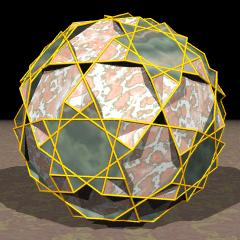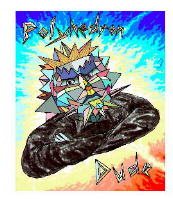Acronym quitdid TOCID symbol tED* Name quasitruncated dodecadodecahedron,stellatruncated dodecadodecahedron `© ©` Circumradius sqrt(11)/2 = 1.658312 Vertex figure [10/3,4,10] General of army f3x5x Colonel of regiment (is itself locally convex – no other uniform polyhedral members) Dihedral angles between {4} and {10/3}:   arccos(-sqrt[(5+sqrt(5))/10]) = 148.282526° between {10} and {10/3}:   arccos(1/sqrt(5)) = 63.434949° between {4} and {10}:   arccos(sqrt[(5-sqrt(5))/10]) = 58.282526° ExternallinksAs abstract polytope quitdid is automorph, thereby interchanging the roles of decagons and decagrams.

Incidence matrix according to Dynkin symbol

```x5/3x5x

.   . . | 120 |  1  1  1 |  1  1  1
--------+-----+----------+---------
x   . . |   2 | 60  *  * |  1  1  0
.   x . |   2 |  * 60  * |  1  0  1
.   . x |   2 |  *  * 60 |  0  1  1
--------+-----+----------+---------
x5/3x . |  10 |  5  5  0 | 12  *  *
x   . x |   4 |  2  0  2 |  * 30  *
.   x5x |  10 |  0  5  5 |  *  * 12

snubbed forms: s5/3s5s
```